2019-03-14 00:19:27 LieberVater 阅读数 417
• ###### Python入门视频精讲

Python入门视频培训课程以通俗易懂的方式讲解Python核心技术，Python基础，Python入门。适合初学者的教程，让你少走弯路！ 课程内容包括：1.Python简介和安装 、2.第一个Python程序、PyCharm的使用 、3.Python基础、4.函数、5.高级特性、6.面向对象、7.模块、8.异常处理和IO操作、9.访问数据库MySQL。教学全程采用笔记+代码案例的形式讲解，通俗易懂！！！

8189 人正在学习 去看看 汤小洋

# 一、关于PIL库

PIL是一个图像处理经常使用到的库，全名是Python Image Library，其中有一个子库是Image，我们今天就来用一下这个库

PIL可以做很多和图像处理相关的事情:

• 图像归档(Image Archives)。PIL非常适合于图像归档以及图像的批处理任务。你可以使用PIL创建缩略图，转换图像格式，打印图像等等。
• 图像展示(Image Display)。PIL较新的版本支持包括Tk PhotoImage，BitmapImage还有Windows DIB等接口。PIL支持众多的GUI框架接口，可以用于图像展示。
• 图像处理(Image Processing)。PIL包括了基础的图像处理函数，包括对点的处理，使用众多的卷积核(convolution kernels)做过滤(filter),还有颜色空间的转换。PIL库同样支持图像的大小转换，图像旋转，以及任意的仿射变换。PIL还有一些直方图的方法，允许你展示图像的一些统计特性。这个可以用来实现图像的自动对比度增强，还有全局的统计分析等。

# 二、PIL库的简单使用

``````# -*- coding: utf-8 -*-
"""
Created on Tue Mar 12 14:03:00 2019

"""

from PIL import Image
from pylab import *

subplot(121)
axis('off')
imshow(img1)

subplot(122)
axis('off')
imshow(img2)

show()``````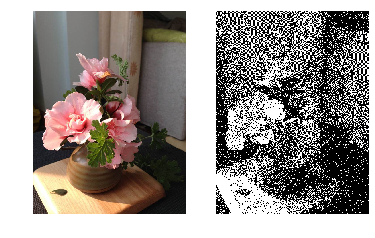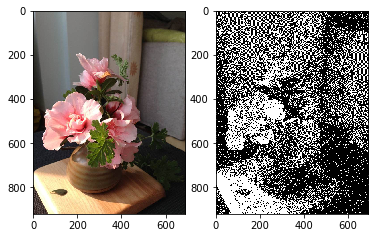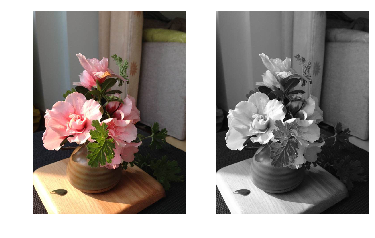L = R * 299/1000 + G * 587/1000+ B * 114/1000

https://www.jianshu.com/p/bdd9bfcbedb7这篇博客中看到了有关convert函数几种模式的介绍，如下：

`convert()`是图像实例对象的一个方法，接受一个 mode 参数，用以指定一种色彩模式
`1 ------------------（1位像素，黑白，每字节一个像素存储）`
`L ------------------（8位像素，黑白）`
`P ------------------（8位像素，使用调色板映射到任何其他模式）`
`RGB------------------（3x8位像素，真彩色）`
`RGBA------------------（4x8位像素，带透明度掩模的真彩色）`
`CMYK--------------------（4x8位像素，分色）`
`YCbCr--------------------（3x8位像素，彩色视频格式）`
`I-----------------------（32位有符号整数像素）`
`F------------------------（32位浮点像素）`

`模式P：`

`￼￼`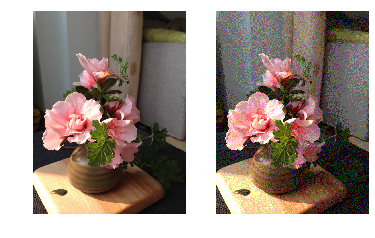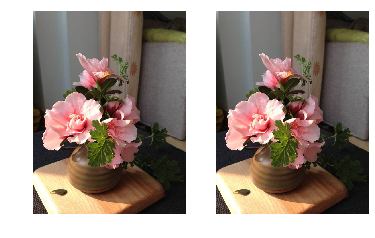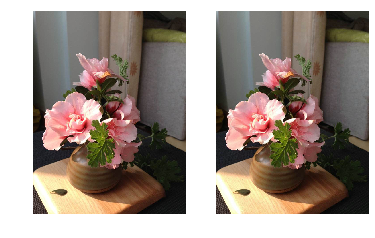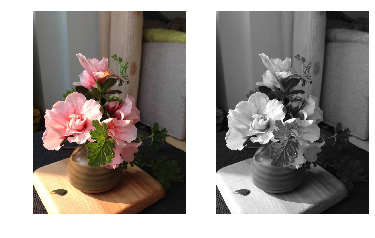python图像处理入门 相关内容

2017-11-26 11:20:01 thatluck 阅读数 271
• ###### Python入门视频精讲

Python入门视频培训课程以通俗易懂的方式讲解Python核心技术，Python基础，Python入门。适合初学者的教程，让你少走弯路！ 课程内容包括：1.Python简介和安装 、2.第一个Python程序、PyCharm的使用 、3.Python基础、4.函数、5.高级特性、6.面向对象、7.模块、8.异常处理和IO操作、9.访问数据库MySQL。教学全程采用笔记+代码案例的形式讲解，通俗易懂！！！

8189 人正在学习 去看看 汤小洋

1.转换成灰度图片

``````from PIL import Image
Image.open('in.jpg').convert('L').save('out.jpg');
``````

2.图形复制与粘贴

``````from PIL import Image
import os

pil_im = Image.open('in.png');
box = (0,162,30,192)
box2 = (200,162,230,192)
region = pil_im.crop(box)
pil_im.paste(region,box2)
pil_im.save('out.png')``````

python图像处理入门 相关内容

2019-03-23 15:38:35 LieberVater 阅读数 193
• ###### Python入门视频精讲

Python入门视频培训课程以通俗易懂的方式讲解Python核心技术，Python基础，Python入门。适合初学者的教程，让你少走弯路！ 课程内容包括：1.Python简介和安装 、2.第一个Python程序、PyCharm的使用 、3.Python基础、4.函数、5.高级特性、6.面向对象、7.模块、8.异常处理和IO操作、9.访问数据库MySQL。教学全程采用笔记+代码案例的形式讲解，通俗易懂！！！

8189 人正在学习 去看看 汤小洋

# 一、获取摄像头

``````cap = cv2.VideoCapture(0)
``````

``face_cascade = cv2.CascadeClassifier(r'haarcascade_frontalface_default.xml') ``

``````face_cascade.load('E:\\anaconda\\pkgs\\libopencv-3.4.1-h875b8b8_3\\Library\\etc\\haarcascades\\haarcascade_frontalface_default.xml')
``````

# 二、后续处理

``````ret, frame = cap.read()
gray = cv2.cvtColor(frame, cv2.COLOR_BGR2GRAY) ``````

# 三、遇到的问题

error: (-215) scn == 3 || scn == 4 in function cv::cvtColor

# 四、程序

``````# -*- coding: utf-8 -*-
"""
Created on Sat Mar 23 14:43:48 2019

"""

import cv2
cap = cv2.VideoCapture(0)
while(True):
if ret is True:
gray = cv2.cvtColor(frame, cv2.COLOR_BGR2GRAY)

else:

break

faces = face_cascade.detectMultiScale(gray, scaleFactor = 1.15, minNeighbors = 5, minSize = (5, 5))
for(x, y, w, h) in faces:
cv2.rectangle(gray, (x, y), (x + w, y + h), (0, 0, 255), 2)

cv2.imshow('Face Recognition', gray)
if cv2.waitKey(1) & 0xFF == ord('q'):
break
cap.release()
cv2.destroyAllWindows()

``````

# 五、效果（马赛克版）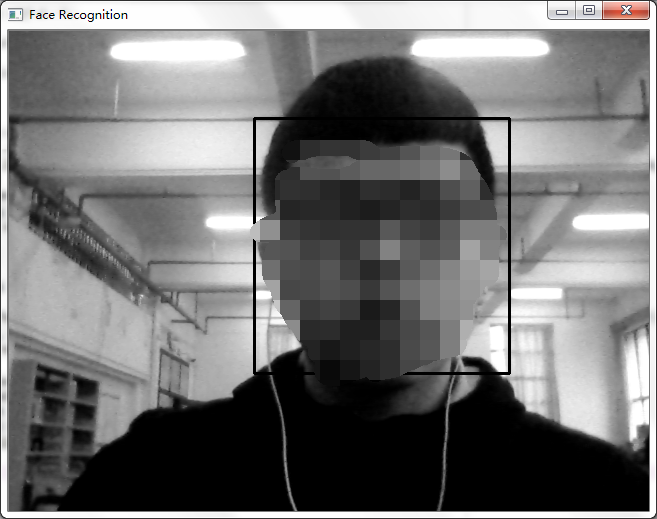python图像处理入门 相关内容

2019-03-13 22:06:01 LieberVater 阅读数 1639
• ###### Python入门视频精讲

Python入门视频培训课程以通俗易懂的方式讲解Python核心技术，Python基础，Python入门。适合初学者的教程，让你少走弯路！ 课程内容包括：1.Python简介和安装 、2.第一个Python程序、PyCharm的使用 、3.Python基础、4.函数、5.高级特性、6.面向对象、7.模块、8.异常处理和IO操作、9.访问数据库MySQL。教学全程采用笔记+代码案例的形式讲解，通俗易懂！！！

8189 人正在学习 去看看 汤小洋

# 一、环境

anaconda有两种下载途径，第一种是从官网下载，第二种是使用清华镜像，清华镜像要快一点，但是一个问题是pycharm使用的时候会报错，这个时候要修改一个.condarc文件，后来又出了各种问题我就想那还是用国外的下载吧，慢就慢一点呗，意外的是其实也没有很慢，这个时候又出了一个错误，这时候重新使用国外下载的版本会报另外一个错误，有些博客提到使用conda update 或者conda update --reset这两条指令，由于前面修改了.condarc文件，所以并没有解决我的问题。可以采用两种方法解决，第一种是改回原来的样子，但是如果像我一样忘了原来的文件怎么办，就可以用第二种方法，将这个文件删掉，再重新执行conda update，成功了。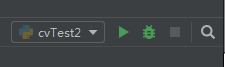我这里对应到cvTest2的下拉框，找到Edit Configuration，修改其中使用的解释器，不然的话由于原来的python解释器和现在的anaconda解释器不同，仍然会提示路径错误，找不到python

Spyder是什么呢？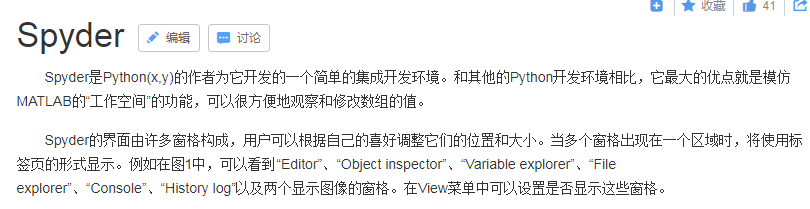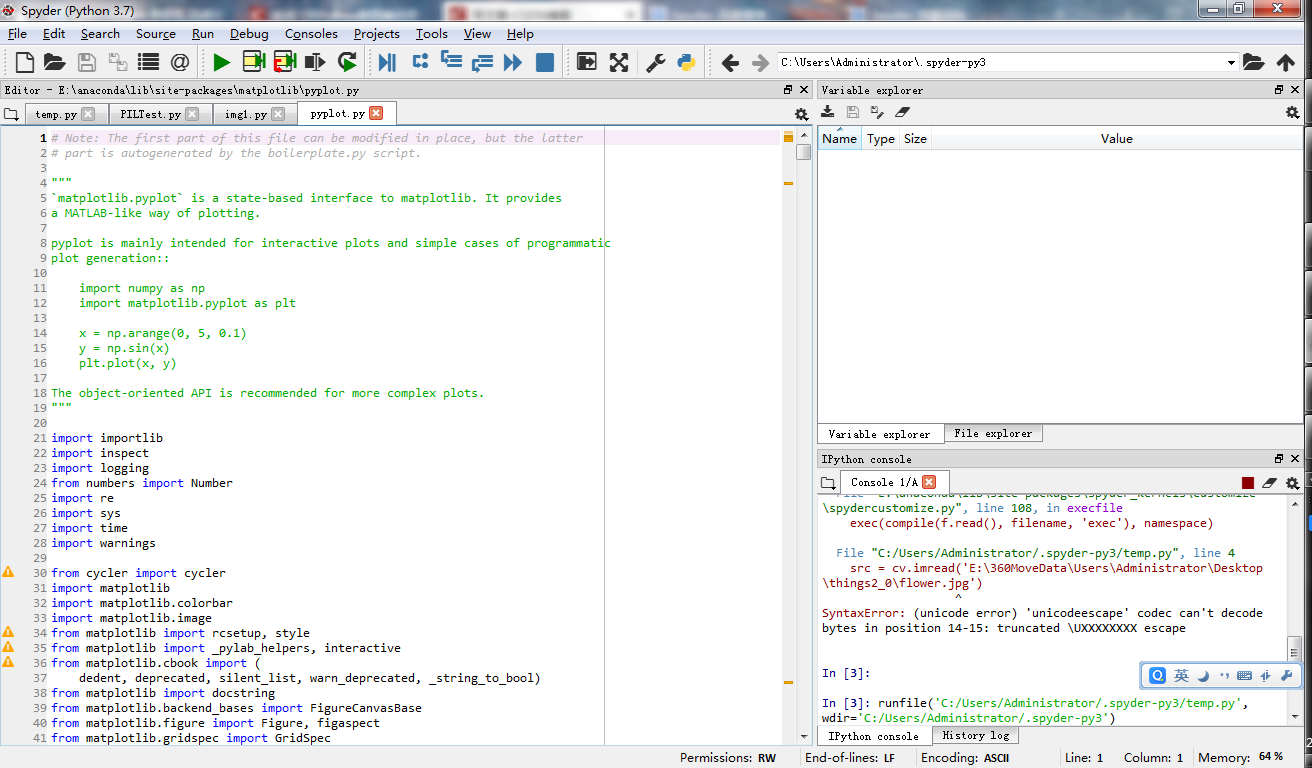# 二、实例——读取一个图片

opencv这个库提供了很多有关图像处理的方法，在这里我们首先执行一个可以读取图片的程序：

``````import cv2 as cv

# 读入图片文件
# 创建一个名字加 “ input image ” 的窗口，
# 窗口可以根据图片大小自动调整
cv.namedWindow('input image', cv.WINDOW_AUTOSIZE)
# 在窗口显示图片
cv.imshow('input image', src)

# 等待用户操作
cv.waitKey(0)
# 释放所有窗口
cv.destroyAllWindows()

``````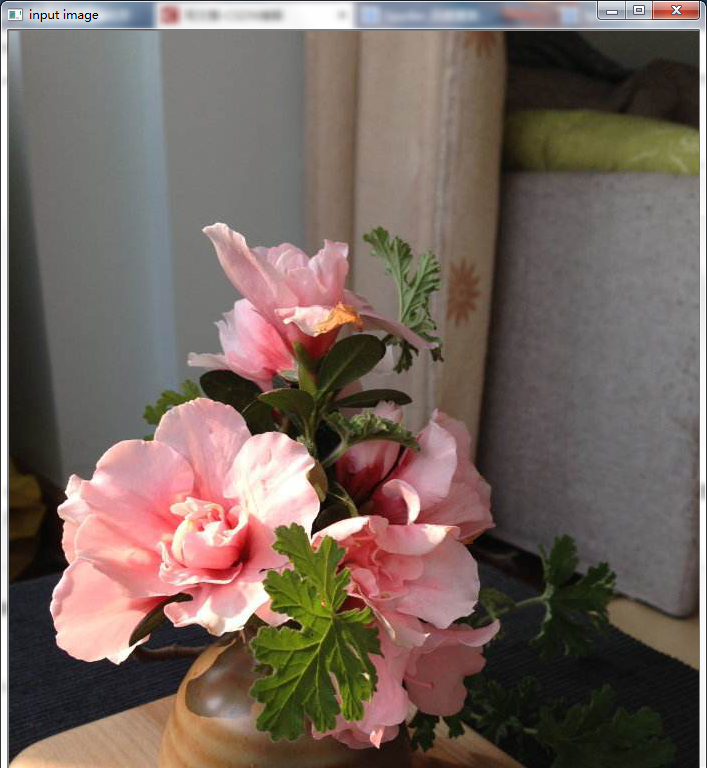python图像处理入门 相关内容

2019-04-18 22:00:00 fendouaini 阅读数 844
• ###### Python入门视频精讲

Python入门视频培训课程以通俗易懂的方式讲解Python核心技术，Python基础，Python入门。适合初学者的教程，让你少走弯路！ 课程内容包括：1.Python简介和安装 、2.第一个Python程序、PyCharm的使用 、3.Python基础、4.函数、5.高级特性、6.面向对象、7.模块、8.异常处理和IO操作、9.访问数据库MySQL。教学全程采用笔记+代码案例的形式讲解，通俗易懂！！！

8189 人正在学习 去看看 汤小洋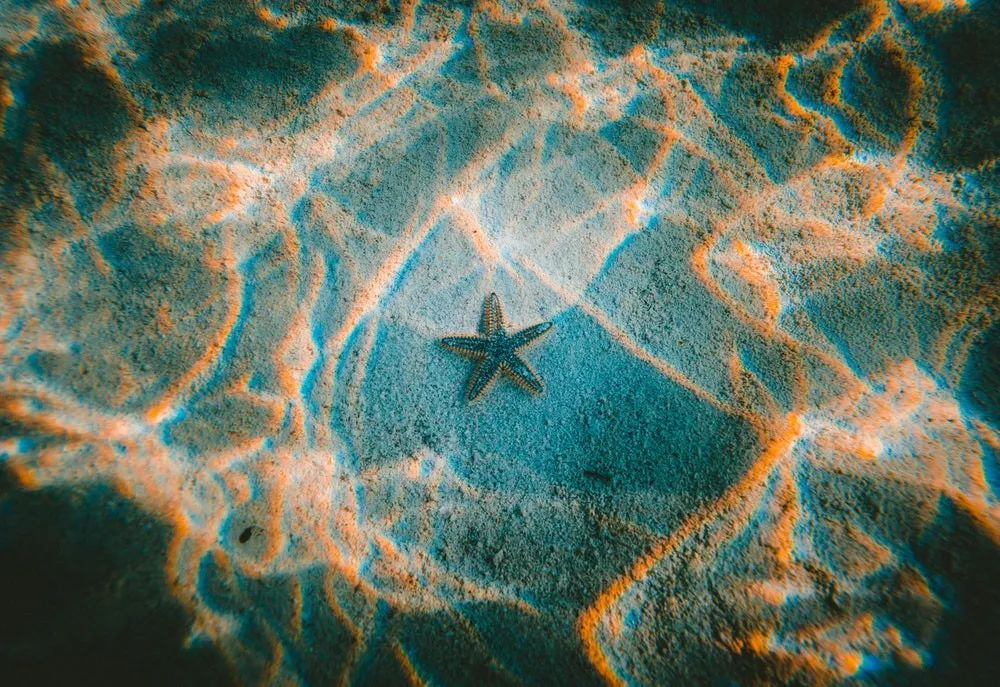【前言】图像预处理对于整个图像处理任务来讲特别重要。如果我们没有进行恰当的预处理，无论我们有多么好的数据也很难得到理想的结果。

1. 理解颜色模型与在图像上绘制图形（图像处理基本操作）。

2. 基本的图像处理与滤波技术。

3. 从特征检测到人脸检测。

4. 图像分割与分水岭（Watershed）算法（TBU）

### 模糊化（Blurring）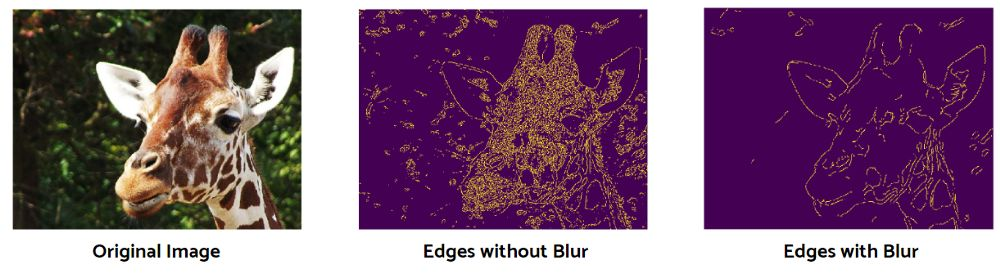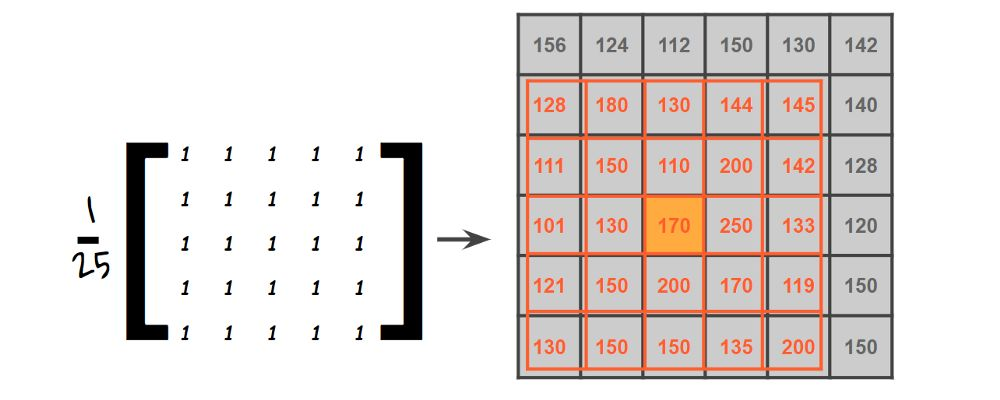``````# Import the image and convert to RGB
img = cv2.cvtColor(img, cv2.COLOR_BGR2RGB)
# Plot the image with different kernel sizes
kernels = [5, 11, 17]
fig, axs = plt.subplots(nrows = 1, ncols = 3, figsize = (20, 20))
for ind, s in enumerate(kernels):
img_blurred = cv2.blur(img, ksize = (s, s))
ax = axs[ind]
ax.imshow(img_blurred)
ax.axis('off')
plt.show()
``````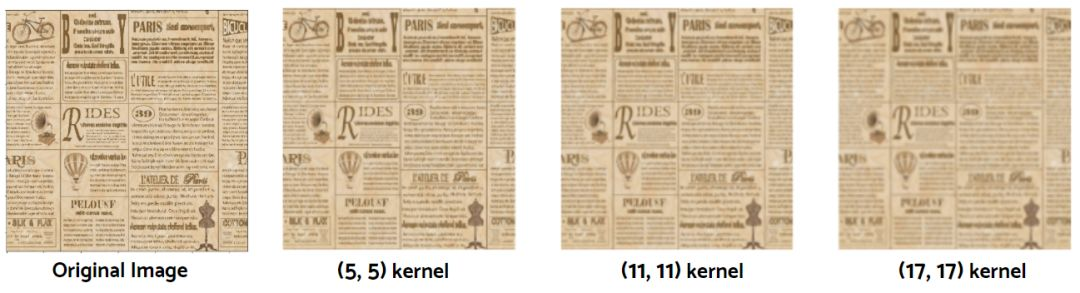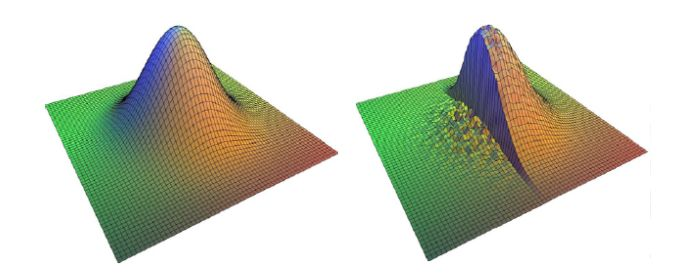``````# Blur the image
img_0 = cv2.blur(img, ksize = (7, 7))
img_1 = cv2.GaussianBlur(img, ksize = (7, 7), sigmaX = 0)
img_2 = cv2.medianBlur(img, 7)
img_3 = cv2.bilateralFilter(img, 7, sigmaSpace = 75, sigmaColor =75)
# Plot the images
images = [img_0, img_1, img_2, img_3]
fig, axs = plt.subplots(nrows = 1, ncols = 4, figsize = (20, 20))
for ind, p in enumerate(images):
ax = axs[ind]
ax.imshow(p)
ax.axis('off')
plt.show()
``````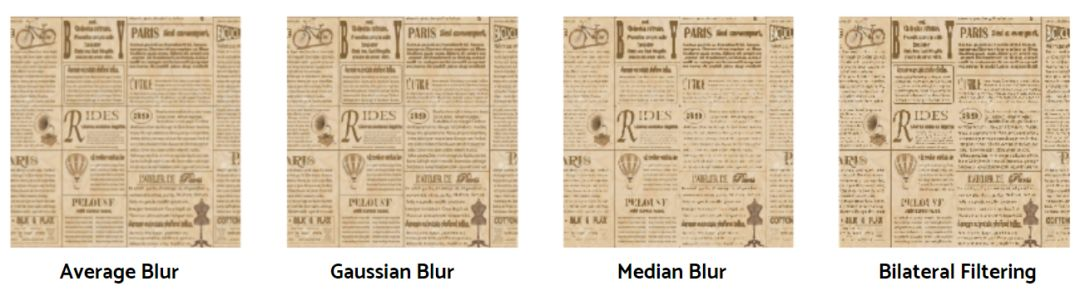## 阈值化（Thresholding）

``````img = cv2.imread('gradation.png')
# Thresholding
_, thresh_0 = cv2.threshold(img, 127, 255, cv2.THRESH_BINARY)
_, thresh_1 = cv2.threshold(img, 127, 255, cv2.THRESH_BINARY_INV)
_, thresh_2 = cv2.threshold(img, 127, 255, cv2.THRESH_TOZERO)
_, thresh_3 = cv2.threshold(img, 127, 255, cv2.THRESH_TOZERO_INV)
_, thresh_4 = cv2.threshold(img, 127, 255, cv2.THRESH_TRUNC)
# Plot the images
images = [img, thresh_0, thresh_1, thresh_2, thresh_3, thresh_4]
fig, axs = plt.subplots(nrows = 2, ncols = 3, figsize = (13, 13))
for ind, p in enumerate(images):
ax = axs[ind//3, ind%3]
ax.imshow(p)
plt.show()
``````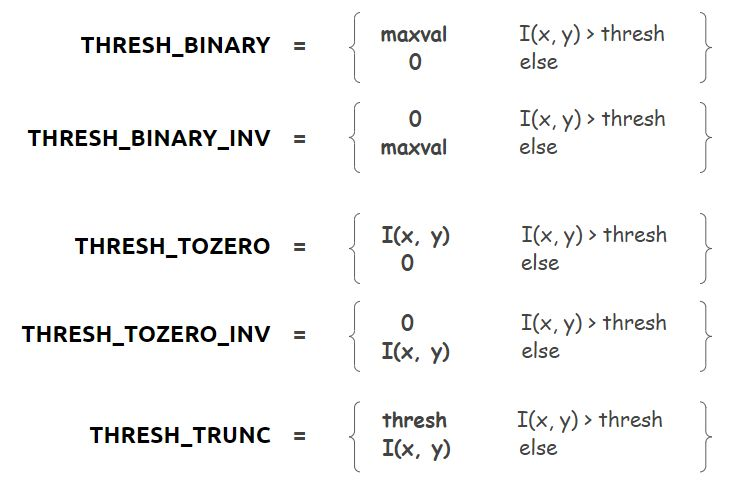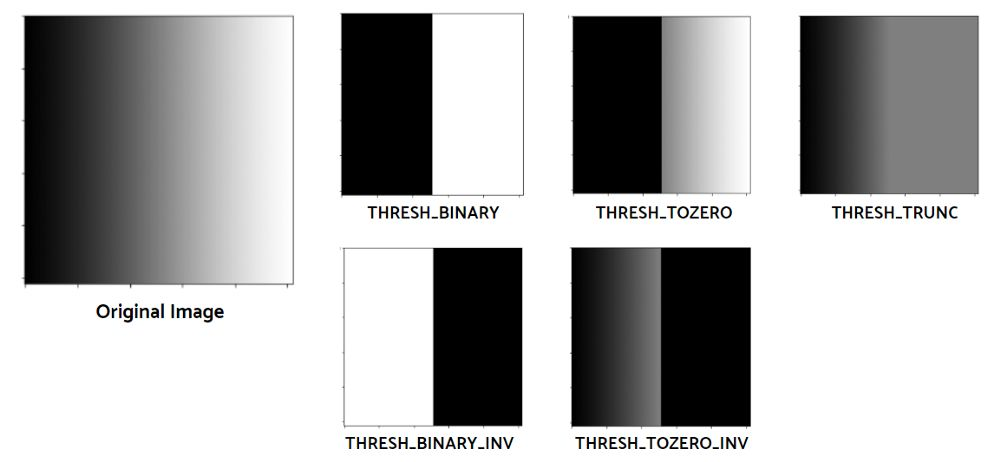ia_10008

``````# Convert the image to grayscale
img = cv2.cvtColor(img, cv2.COLOR_BGR2GRAY)
_, thresh_binary = cv2.threshold(img, thresh = 127, maxval = 255, type = cv2.THRESH_BINARY)
cv2.THRESH_BINARY, 7, 2)
cv2.THRESH_BINARY_INV, 7, 2)
cv2.THRESH_BINARY, 7, 8)
cv2.THRESH_BINARY, 7, 8)
``````

``````# Plot the images
fig, axs = plt.subplots(nrows = 2, ncols = 3, figsize = (15, 15))
for ind, p in enumerate(images):
ax = axs[ind%2, ind//2]
ax.imshow(p, cmap = 'gray')
ax.axis('off')
plt.show()
``````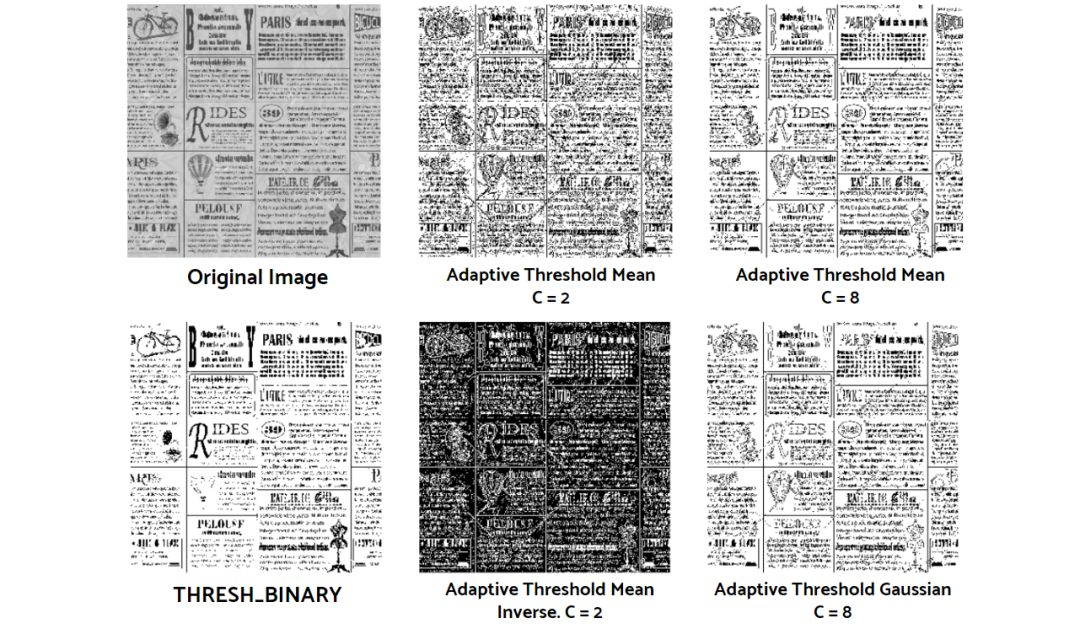``````# Apply gradient filtering
sobel_x = cv2.Sobel(img, cv2.CV_64F, dx = 1, dy = 0, ksize = 5)
sobel_y = cv2.Sobel(img, cv2.CV_64F, dx = 0, dy = 1, ksize = 5)
beta=0.5, gamma=0)
laplacian = cv2.Laplacian(img, cv2.CV_64F)
``````

Sobel运算同时使用高斯平滑和微分。我们通过cv2.Sobel()函数使用它，可以定义两个不同的方向:垂直方向(sobel_x)和水平方向(sobel_y)。dx和dy表示导数。当dx = 1时，通过计算像素值沿水平方向的导数，从而进行图像滤波。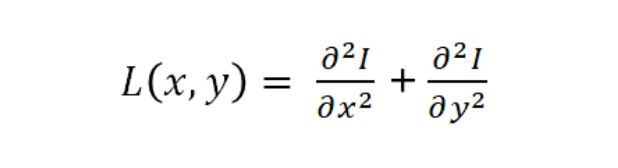``````# Plot the images
images = [sobel_x, sobel_y, blended, laplacian]
plt.figure(figsize = (20, 20))
for i in range(4):
plt.subplot(1, 4, i+1)
plt.imshow(images[i], cmap = 'gray')
plt.axis('off')
plt.show()
``````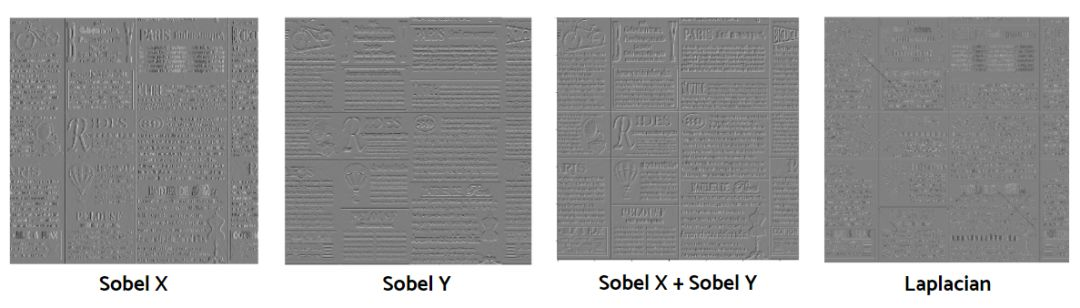## 形态转换（Morpgological transformations）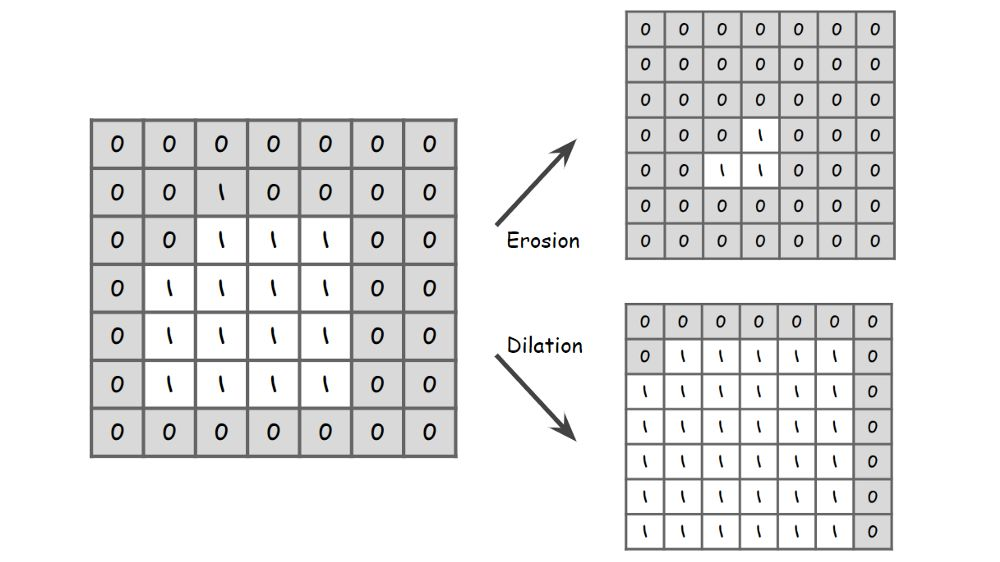``````img = cv2.imread('simpson.jpg')
# Create erosion kernels
kernel_0 = np.ones((9, 9), np.uint8)
kernel_1 = cv2.getStructuringElement(cv2.MORPH_ELLIPSE, (9, 9))
kernel_2 = cv2.getStructuringElement(cv2.MORPH_CROSS, (9, 9))
kernels = [kernel_0, kernel_1, kernel_2]
# Plot the images
plt.figure(figsize = (20, 20))
for i in range(3):
img_copy = img.copy()
img_copy = cv2.erode(img_copy, kernels[i], iterations = 3)
plt.subplot(1, 3, i+1)
plt.imshow(img_copy)
plt.axis('off')
plt.show()
``````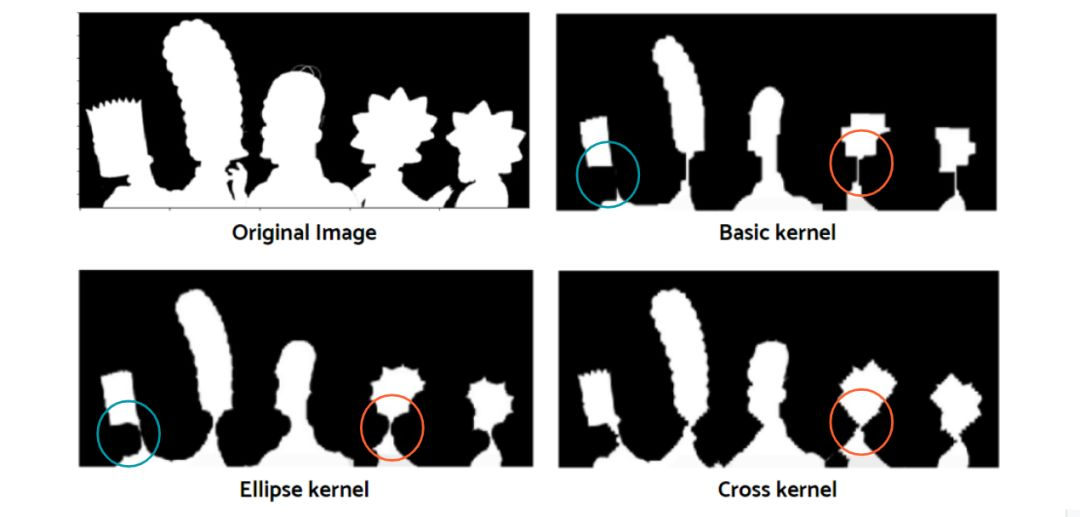``````# Apply dilation
kernel = np.ones((9, 9), np.uint8)
img_dilate = cv2.dilate(img, kernel, iterations = 3)
plt.figure(figsize = (20, 10))
plt.subplot(1, 2, 1); plt.imshow(img, cmap="gray")
plt.subplot(1, 2, 2); plt.imshow(img_dilate, cmap="gray")
plt.show()
``````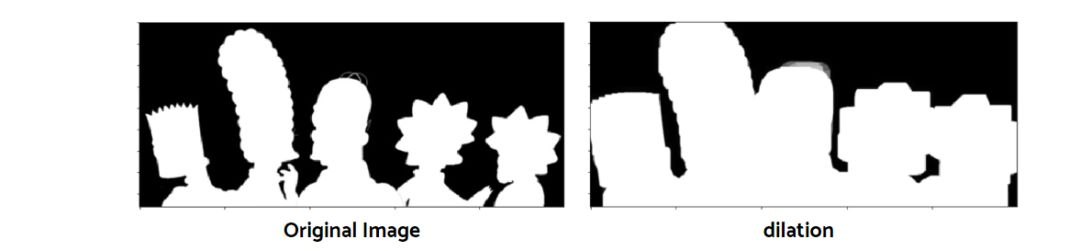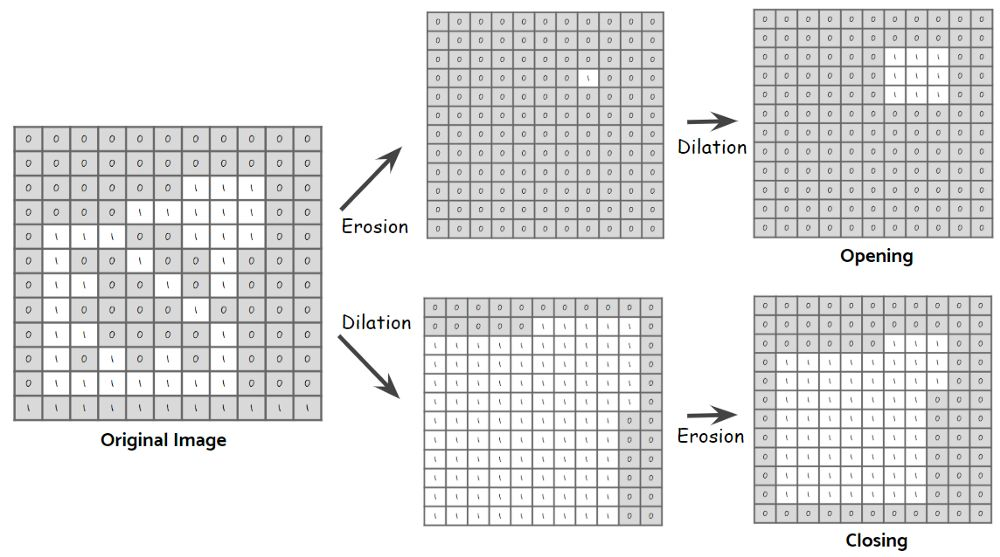``````# Apply the operations
kernel = np.ones((9, 9), np.uint8)
img_open = cv2.morphologyEx(img, op= cv2.MORPH_OPEN, kernel)
img_close = cv2.morphologyEx(img, op= cv2.MORPH_CLOSE, kernel)
img_tophat = cv2.morphologyEx(img, op= cv2.MORPH_TOPHAT, kernel)
img_blackhat = cv2.morphologyEx(img, op= cv2.MORPH_BLACKHAT, kernel)
# Plot the images
images = [img, img_open, img_close, img_grad,
img_tophat, img_blackhat]
fig, axs = plt.subplots(nrows = 2, ncols = 3, figsize = (15, 15))
for ind, p in enumerate(images):
ax = axs[ind//3, ind%3]
ax.imshow(p, cmap = 'gray')
ax.axis('off')
plt.show()
``````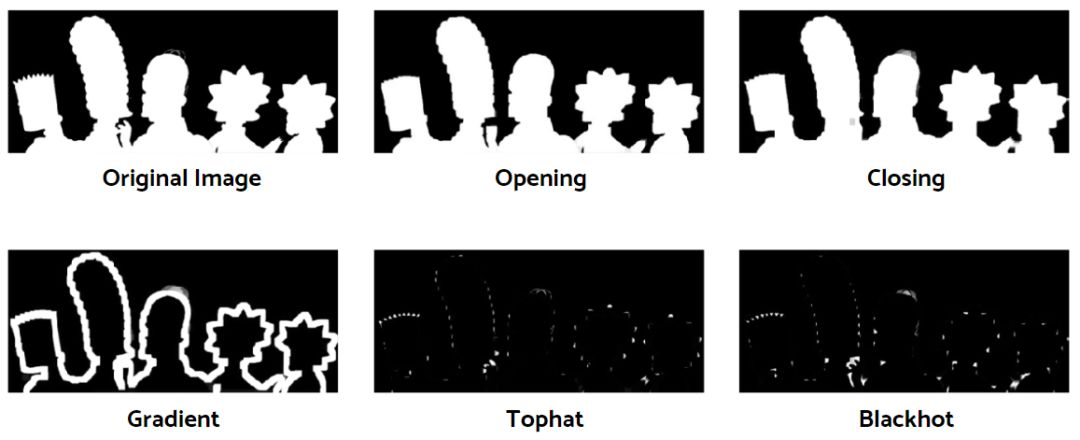## 总结与展望

http://docs.panchuang.net/

http://pytorch.panchuang.net/

python图像处理入门 相关内容